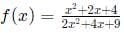CAT  >  CAT Previous Year Questions - Functions

# CAT Previous Year Questions - Functions Notes | Study Quantitative Aptitude (Quant) - CAT

## Document Description: CAT Previous Year Questions - Functions for CAT 2022 is part of Quantitative Aptitude (Quant) preparation. The notes and questions for CAT Previous Year Questions - Functions have been prepared according to the CAT exam syllabus. Information about CAT Previous Year Questions - Functions covers topics like and CAT Previous Year Questions - Functions Example, for CAT 2022 Exam. Find important definitions, questions, notes, meanings, examples, exercises and tests below for CAT Previous Year Questions - Functions.

Introduction of CAT Previous Year Questions - Functions in English is available as part of our Quantitative Aptitude (Quant) for CAT & CAT Previous Year Questions - Functions in Hindi for Quantitative Aptitude (Quant) course. Download more important topics related with notes, lectures and mock test series for CAT Exam by signing up for free. CAT: CAT Previous Year Questions - Functions Notes | Study Quantitative Aptitude (Quant) - CAT
 1 Crore+ students have signed up on EduRev. Have you?

Question for CAT Previous Year Questions - Functions
Try yourself:For all real values of x, the range of the functionis



Question for CAT Previous Year Questions - Functions
Try yourself:If x2 − 7x and g(x) = x + 3g(x) = x + 3, then the minimum value of f(g(x)) - 3xf(g(x)) − 3x is:



Question for CAT Previous Year Questions - Functions
Try yourself:If f ( x + y ) = f ( x) f ( y) and f (5) = 4, then f (10) - f (-10) is equal to



*Answer can only contain numeric values
Question for CAT Previous Year Questions - Functions
Try yourself:For any positive integer n, let f(n) = n(n + 1) if n is even, and f(n) = n + 3 if n is odd. If m is a positive integer such that 8f(m + 1) - f(m) = 2, then m equals

[2019 TITA]

*Answer can only contain numeric values
Question for CAT Previous Year Questions - Functions
Try yourself:Consider a function f(x + y) = f(x) f(y) where x , y are positive integers, and f(1) = 2. If f (a + 1) + f (a + 2) + ..... + f(a + n) = 16 (2n - 1) then a is equal to.

[2019 TITA]

*Answer can only contain numeric values
Question for CAT Previous Year Questions - Functions
Try yourself:Let f be a function such that f (mn) = f (m) f (n) for every positive integers m and n. If f (1), f (2) and f (3) are positive integers, f (1) < f (2), and f (24) = 54, then f (18) equals

[2019 TITA]

*Answer can only contain numeric values
Question for CAT Previous Year Questions - Functions
Try yourself:If f(x + 2) = f(x) + f(x + 1) for all positive integers x, and f(11) = 91, f(15) = 617, then f(10) equals.

[2018 TITA]

*Answer can only contain numeric values
Question for CAT Previous Year Questions - Functions
Try yourself:Let f(x) = min{2x2, 52 - 5x}, where x is any positive real number.Then the maximum possible value of f(x) is

[2018 TITA]

*Answer can only contain numeric values
Question for CAT Previous Year Questions - Functions
Try yourself:Let f(x) = max{5x, 52 - 2x2}, where x is any positive real number.Then the minimum possible value of f(x) is

[2018 TITA]

*Answer can only contain numeric values
Question for CAT Previous Year Questions - Functions
Try yourself:If f1(x) = x2 + 11x + n and f2(x) = x, then the largest positive integer n for which the equation f1(x) = f2(x) has two distinct real roots, is:

[2017 TITA]

Question for CAT Previous Year Questions - Functions
Try yourself:Let f(x) = 2x – 5 and g(x) = 7 – 2x. Then |f(x) + g(x)| = |f(x)| + |g(x)| if and only if



Question for CAT Previous Year Questions - Functions
Try yourself:If f(x) = 5x + 2 / 3x − 5 and g(x) = x2 – 2x – 1, then the value of g(f(f(3))) is:



Question for CAT Previous Year Questions - Functions
Try yourself:Let f(x) = x2 and g(x) = 2x, for all real x. Then the value of f(f(g(x)) + g(f(x))) at x = 1 is



*Answer can only contain numeric values
Question for CAT Previous Year Questions - Functions
Try yourself:If f(ab) = f(a)f(b) for all positive integers a and b, then the largest possible value of f(1) is

[2017 TITA]

The document CAT Previous Year Questions - Functions Notes | Study Quantitative Aptitude (Quant) - CAT is a part of the CAT Course Quantitative Aptitude (Quant).
All you need of CAT at this link: CAT

## Quantitative Aptitude (Quant)

163 videos|164 docs|131 tests
 Use Code STAYHOME200 and get INR 200 additional OFF

## Quantitative Aptitude (Quant)

163 videos|164 docs|131 tests

### How to Prepare for CAT

Read our guide to prepare for CAT which is created by Toppers & the best Teachers

Track your progress, build streaks, highlight & save important lessons and more!

,

,

,

,

,

,

,

,

,

,

,

,

,

,

,

,

,

,

,

,

,

;